### Special Relativity

Having unlocked the Lorentz transformation in the last quiz, we can now take the coordinates of an event in one inertial reference frame, and calculate the coordinates of the same event in a different inertial frame, provided we know the relative velocity between the frames.

In the rest of this chapter, we will use the Lorentz transformation to clarify some results that, up to this point, we haven't been able to explain completely. For instance, why don't Michelson and Morley detect a time difference along perpendicular arms of their interferometer?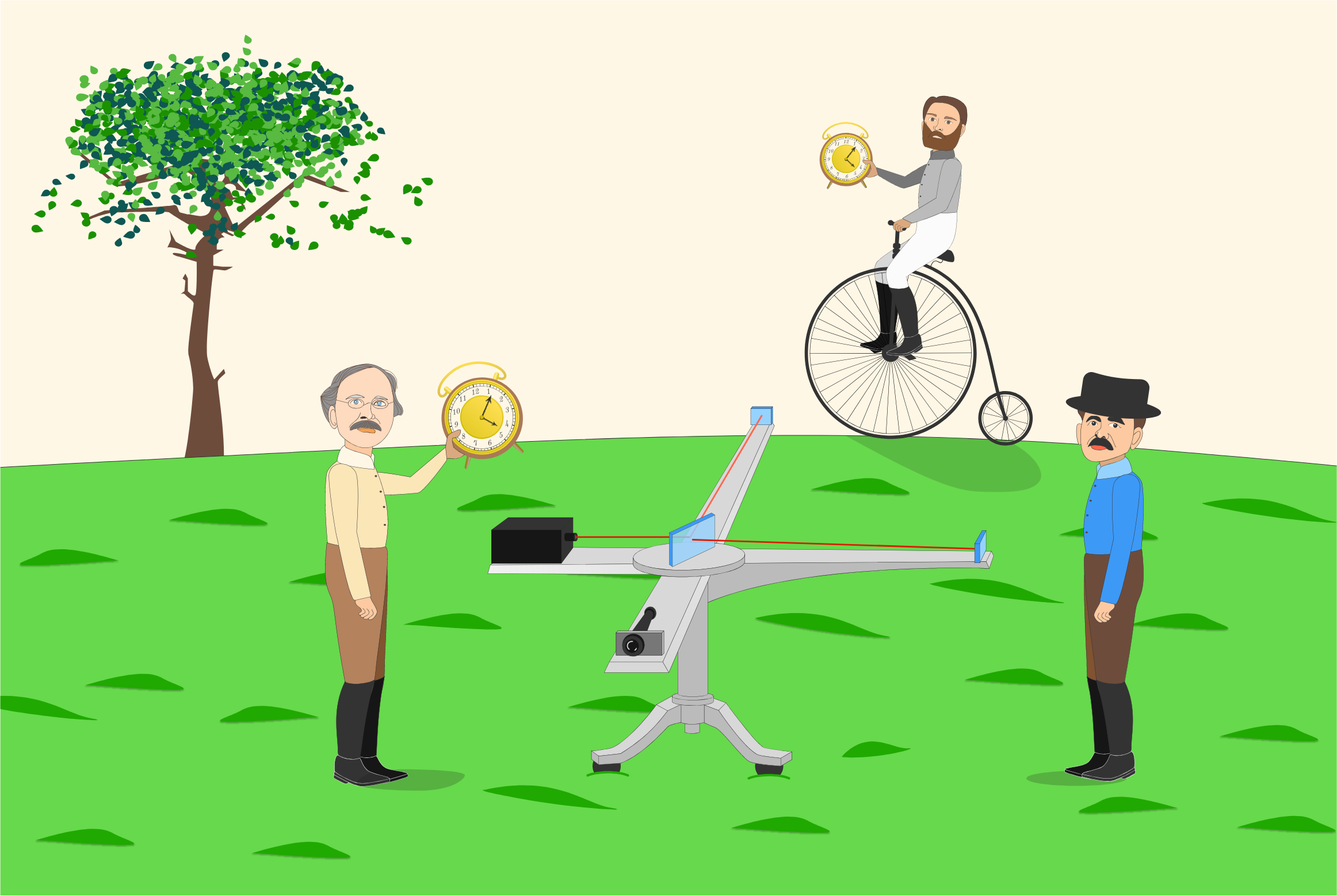In this quiz, you're helping them re-analyze their experiment under Einstein's postulates. They have lent you one of their bikes and handed you a clock, so that you can make measurements from a frame moving with relative speed $V$ while they operate the experiment in their frame.

# Length Contraction & Time Dilation

You now know that a Lorentz transformation mixes time and space coordinates when you move into a different frame, and Morley suspects this may affect the time measured by a moving clock. Before firing up the interferometer's lasers, Morley wants to do a test.

What Morley suggests is simple. He will start with both his clock and your clock at his origin $X=0.$ Then, at a time he designates $T=0,$ he will throw your clock with speed $V$ while you stand a distance of $X=D$ away. You'll catch it, immediately read off the elapsed time, and compare it to the time on Morley's clock, which hasn't moved.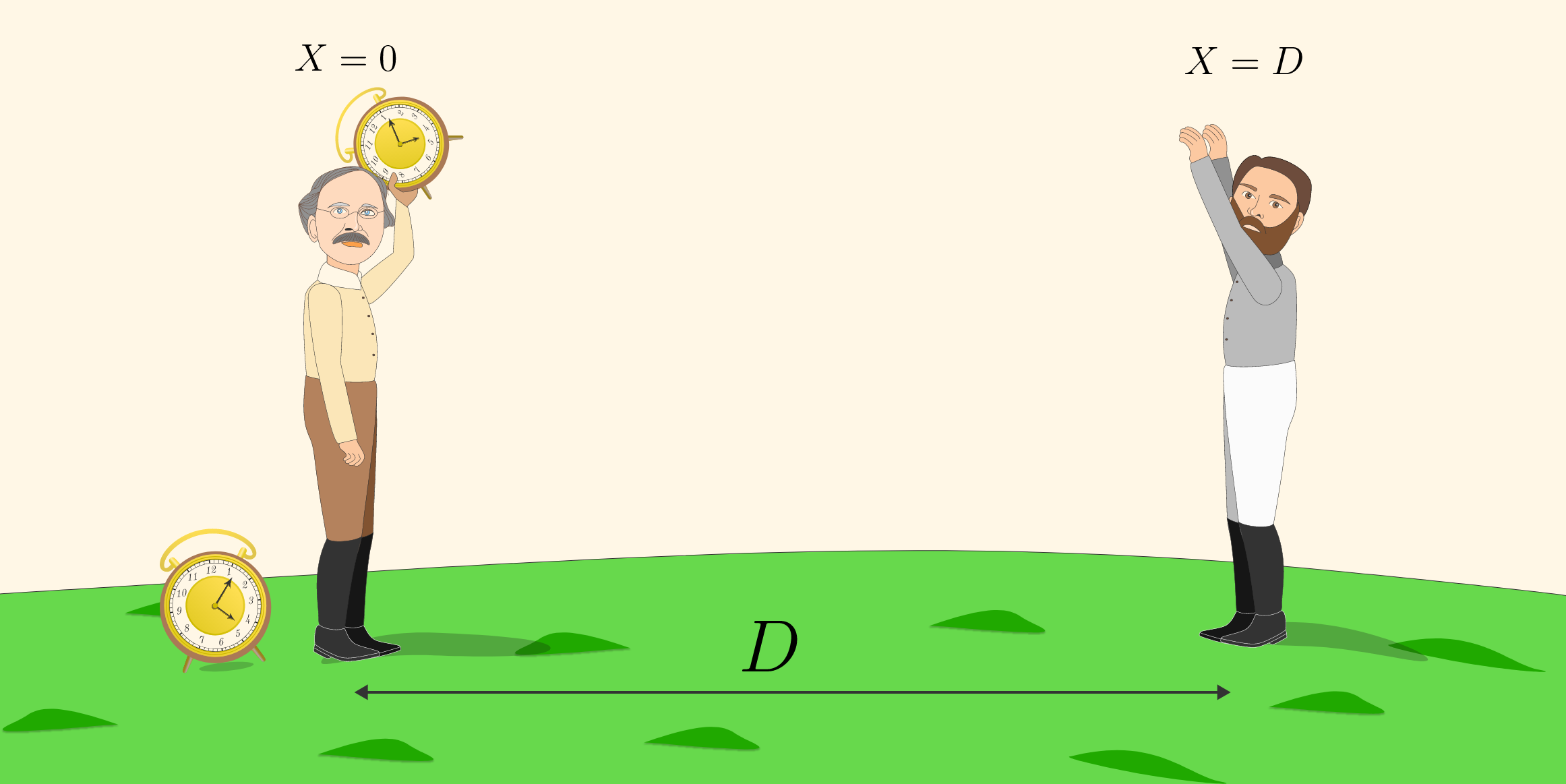In this quiz, we will ignore all accelerations, including gravity and the clock's acceleration as Morley gets ready to throw. In a later chapter, we will look specifically at accelerating clocks.

# Length Contraction & Time Dilation

Each clock is measuring the time between two events in its own reference frame:

• Event 1: Morley throws the clock.
• Event 2: You catch the clock.

A Lorentz transformation relates the coordinates of these events in each clock's reference frame. You decide to call the clock's frame the primed coordinates, and the unprimed coordinates are Morley's. Morley picks his $X$-axis in the same direction of the throw, which aligns with the clock's $X^\prime$-axis.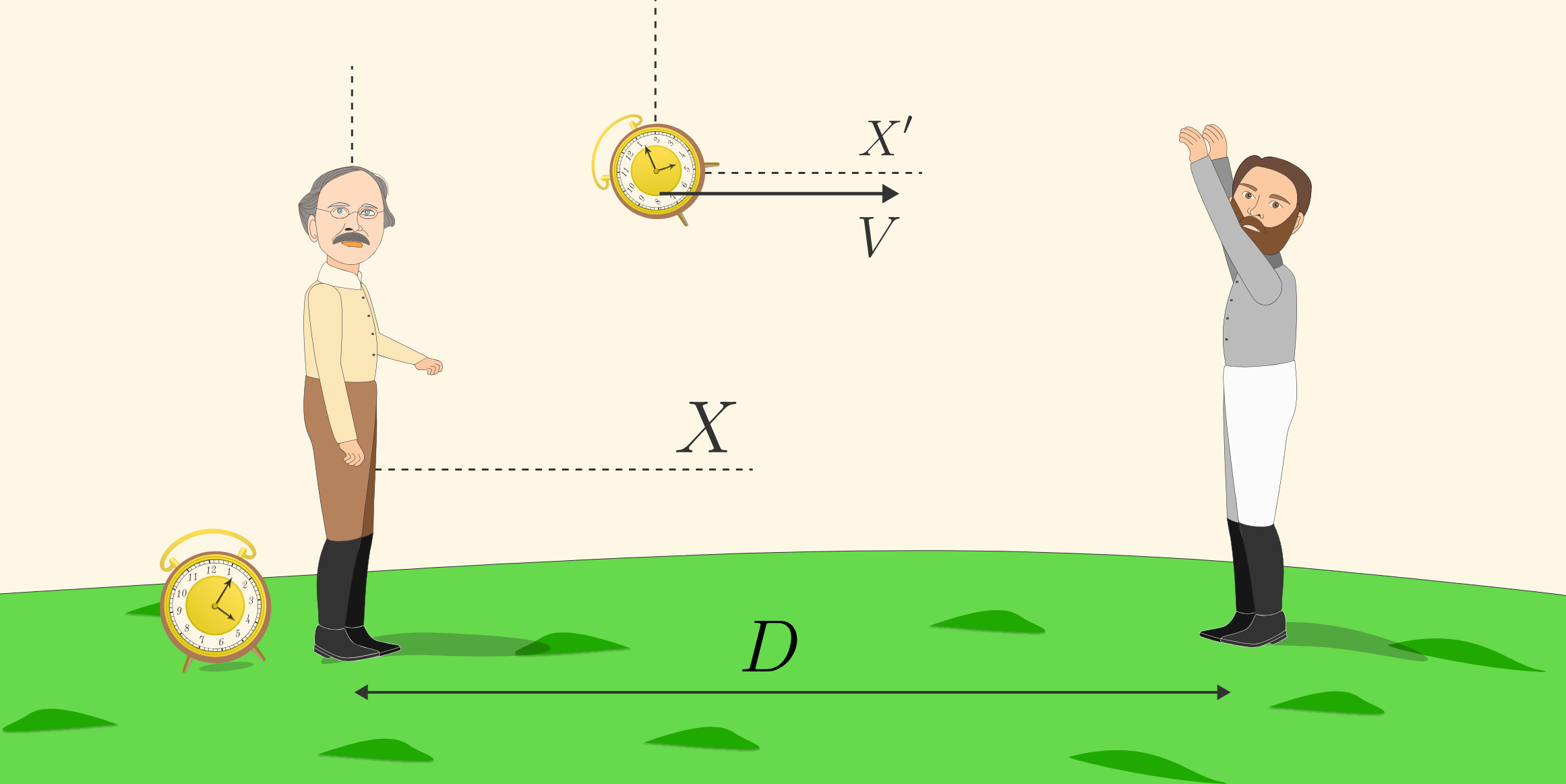The coordinates of Event 1 in yours and Morley's frame are $(T=0,X=0,Y=0,Z=0).$ Because the clock moves only along the $X$-axis, and the $Y$- and $Z$-coordinates remain zero, we will abbreviate the coordinates of this event as $(0,0),$ with the understanding that the first coordinate listed is $T.$

What are the coordinates of Event 2 in yours and Morley's reference frame?

# Length Contraction & Time Dilation

In the last quiz, you derived the Lorentz transformation between two reference frames moving relative to each other along the $x$-direction: \begin{aligned} x^\prime &= \gamma \left(x-vt \right) \\ t^\prime &= \gamma \left(t - \frac{v}{c^2} x\right). \end{aligned}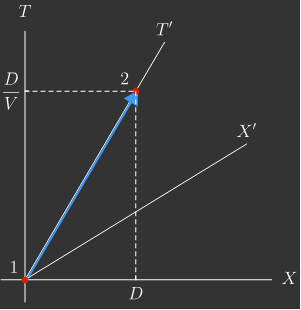In the thrown clock's frame, the coordinates of Event $1$ remain $(0,0).$ Use the Lorentz transformation to find the time coordinate $T^\prime$ of Event $2.$

At the instant you catch the moving clock, what should it read?

Hint: You will be able to simplify your answer by noting that $1-\frac{V^2}{c^2} = \frac{1}{\gamma^2}.$

# Length Contraction & Time Dilation

Which is less: the time $T^\prime$ measured by the thrown clock, or the time $T$ measured by the clock in your frame?

Recall: The Lorentz factor is defined by $\gamma = \dfrac1{\sqrt{1-\frac{V^2}{c^2}}}.$

# Length Contraction & Time Dilation

We have just encountered the relativistic effect of time dilation:

In other words, a clock attached to the interferometer would log less time compared to a clock that you're holding. In fact, the moving clock would tick slower. The time $T^\prime$ that ticks by on a clock moving relative to you is related to the time $T$ that passes on your clock by $T^\prime = T/\gamma.$

This effect is built into the Lorentz transformations. Time dilation is not an effect of seeing a moving clock; in fact, a clock moving relative to you ticks slower (whether you're watching it or not).

This is one of the most surprising (and most thoroughly tested) results in Einstein's theory. One of the most notable tests took place in the 1970's in which scientists equipped commercial airlines with high-precision atomic clocks as they trotted across the globe. As predicted by relativity, the clocks measured less time while in flight compared to clocks on the ground.

# Length Contraction & Time Dilation

Suddenly, Michelson pipes up and poses a question: if observers in different frames measure different times between events, could they also measure different distances too?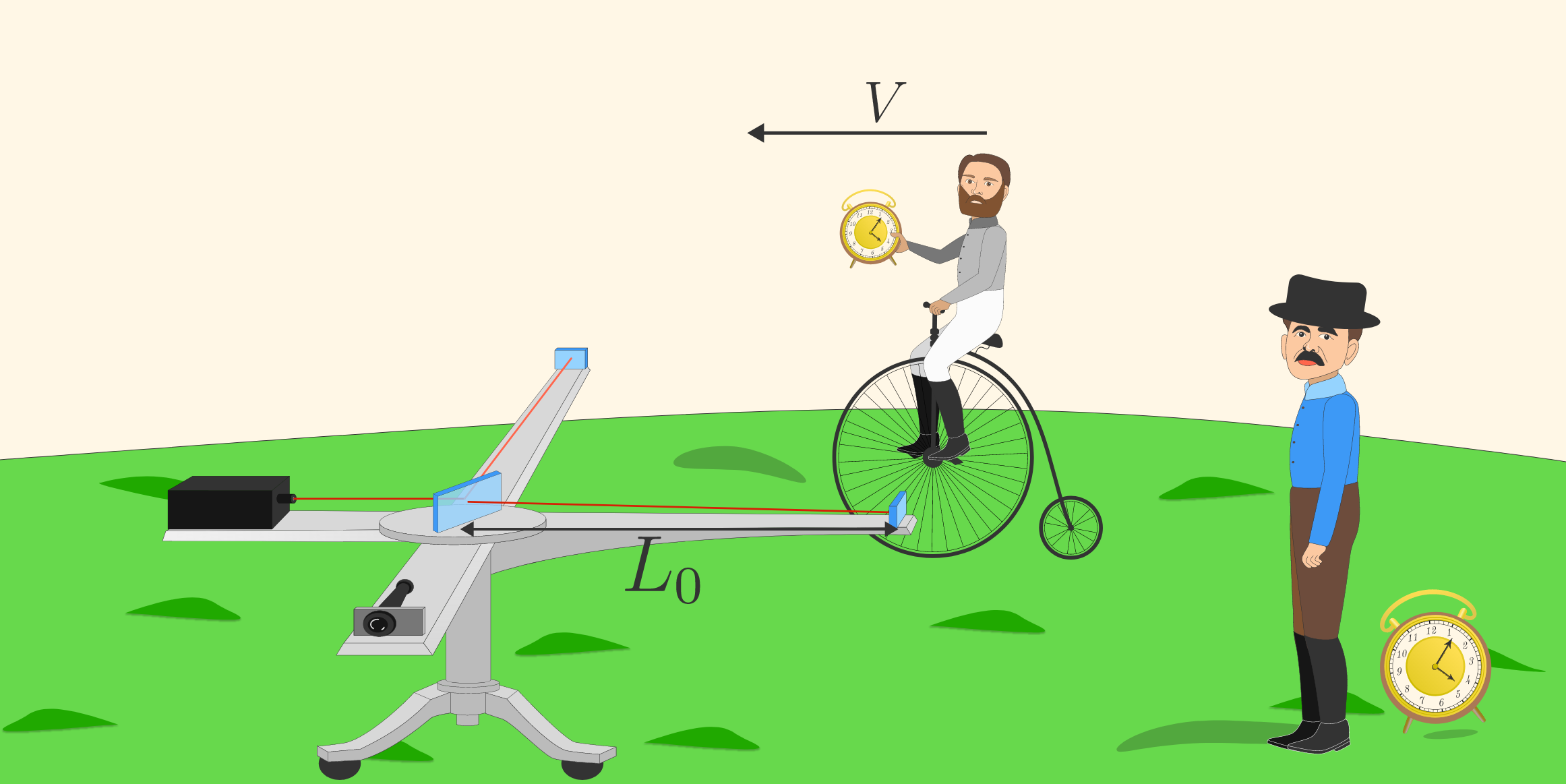To answer this, Michelson wants you to measure the length of the interferometer's arm as you pedal past on your bike. It's not so easy to use a meterstick while biking, so he suggests you measure its length by timing how long it takes you to ride past at a constant speed $V$. He'll use his clock to make a control measurement in the interferometer's reference frame.

In Michelson's frame, the arm of the interferometer has length $L_0,$ so he measures time $\Delta T = \frac{L_0}V$ as you race by.

How much time do you measure in the frame of the bike?

# Length Contraction & Time Dilation

Einstein says that all inertial frames are equivalent, physically speaking, so in the bike's frame, the arm of the interferometer is passing you with speed $V.$

Given that your clock is ticking slower, how far apart are the ends of the interferometer? This is the length of the arm in the bike's frame.

# Length Contraction & Time Dilation

We just found that an object moving past you quickly is shorter than its length at rest. We say "is shorter," not "appears shorter," because this is not just an illusion—in different reference frames lengths are genuinely different. This relativistic effect is called length contraction.

Length contraction is also encoded in the Lorentz transformation. At $T=0$ in your frame, suppose the far end of the arm is at $X=L.$ Using the Lorentz transformation to find $X^\prime=\gamma(X-vT),$ the coordinate of the arm in the interferometer's frame, we have $X^\prime = \gamma L.$ But, of course, in the interferometer's frame, the right end of the arm is always on $X^\prime=L_0,$ so again we find $L=\frac{L_0}{\gamma}.$

Interestingly, since $Y^\prime=Y$ and $Z^\prime=Z,$ length contraction only occurs along the direction of relative motion $($which we have been calling $X).$

# Length Contraction & Time Dilation

In the Luminiferous Aether quiz, we predicted that someone moving relative to an interferometer would measure a propagation time difference $\Delta T$ between the arms (and would see the beams interfering on the detector).

However, someone in the rest frame of an interferometer would measure no difference and the beams would recombine perfectly aligned.

If all inertial frames are equivalent, how do we resolve these different results? In fact, we now have the tools to show definitively that someone passing the interferometer in an inertial frame would actually measure $\Delta T=0,$ same as someone at rest relative to the interferometer.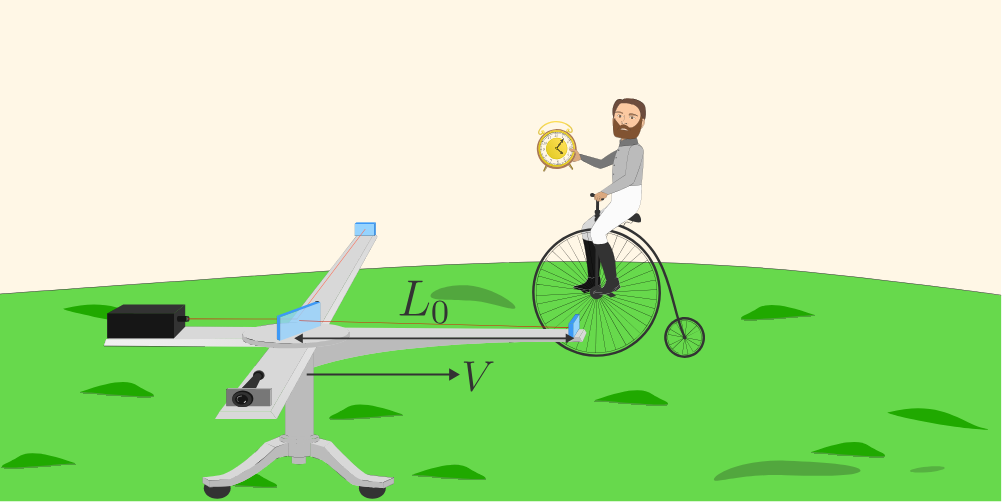Accounting for time dilation and length contraction, how much time does a beam take to propagate from the center, to the end of either arm, and back again, as measured by an observer moving with speed $V?$

Assume that in the rest frame of the interferometer, the arms are the same length $L_0.$

# Length Contraction & Time Dilation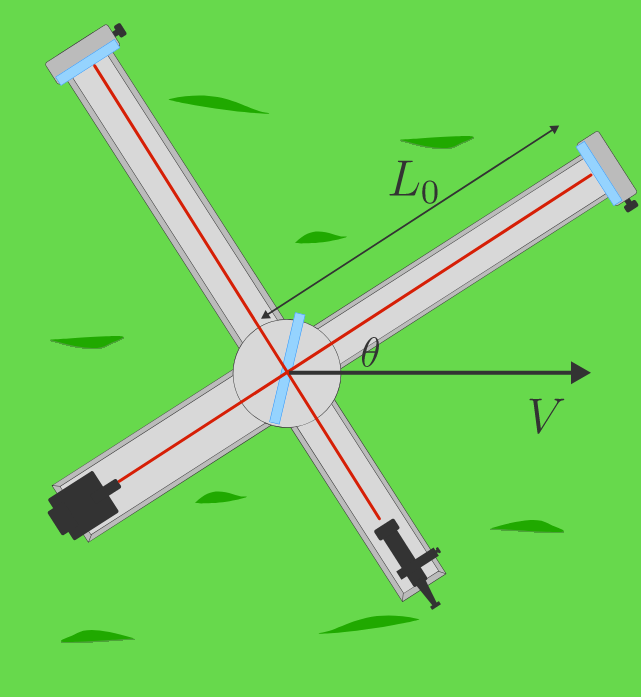Is the result in the previous question true even when one of the arms isn't aligned with the relative velocity? Suppose one arm of the interferometer makes an angle of $\theta$ with the velocity. How long does the beam take to propagate back and forth along each arm in a reference frame moving with speed $V$ relative to the interferometer?

# Length Contraction & Time Dilation

Using the Lorentz transformation, we have validated Michelson and Morley's observation that the beams will take the same amount of time to traverse both arms, no matter how fast it is moving or how it is oriented.

We also discovered two of the most surprising relativistic phenomena: time dilation and length contraction. Outside of the simplest situations, it is not easy to apply these as fundamental principles. Essentially, they are properties of the Lorentz transformation, which you can always fall back on.In the next quiz, we will return to space and see what other relativistic effects we can discover hidden within the Lorentz transformations.

# Length Contraction & Time Dilation

×